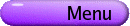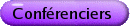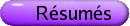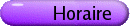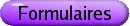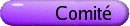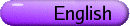Algèbres d'opérateurs, espaces d'opérateurs et analyse harmonique Org: Ken Davidson et Brian Forrest (Waterloo)[PDF] MONICA ILIE, Lakehead University, Thunder Bay, Ontario On completely bounded Fourier algebra homomorphisms [PDF] We present a survey of some recent results regarding completely bounded homomorphisms of the Fourier algebra of a locally compact group G. We discuss the description as well as the w*-w* continuity of such homomorphisms. This talk is based on separate work with Nico Spronk and with Ross Stokke. EBERHARD KANIUTH, University of Paderborn, 33095 Paderborn, Germany Stable rank and real rank of group C*-algebras [PDF] The stable rank and the real rank of a C*-algebra A have been introduced by Rieffel and Brown and Pedersen, respectively, as non-commutative analogues of the complex and the real dimension of topological spaces. In fact, for the algebra of continuous functions on a compact Hausdorff space X one has RR(C(X)) = dimX and a similar formula for sr(C(X)). Both of these ranks (in particular the condition RR(A)=0) have played a significant role in the classification of C*-algebras, and there is now an extensive literature available, especially on group C*-algebras. The talk will focus on recent work with R. J. Archbold (Aberdeen, Scotland) and will discuss formulæ for the ranks of group C*-algebras C*(G) for various classes of locally compact groups G, such as nilpotent groups, motion groups and crystal groups. DAVID KRIBS, University of Guelph On graph algebras and related things [PDF] In this talk I will discuss recent joint work with Elias Katsoulis on the class of operator algebras associated with directed graphs. Time permitting, I will also outline a unified framework for studying both graph algebras and the causal history formalism from quantum gravity. ANTHONY LAU, University of Alberta, Edmonton, AB T6G 2G1 Separation and Extension Properties of Positive Definite Functions on Locally Compact Groups [PDF] Let G be a locally compact group and P(G) be the set of continuous positive definite functions on G, i.e., all continuous functions f: G®C such that Sli[(l)]j f(xi x-1j) ³ 0 for any l1, ...,ln Î C and x1,...,xn Î G. A closed subgroup H of G is called separating if for each x Î G\H, there exists f Î P(G) such that f(x) ¹ 1, and f(h)=1 for all h Î H; H is called extending if for each f Î P(H) there exists [(f)\tilde] Î P(G) such that [(f)\tilde] extends f. G is said to have the separation property (respectively extension property) if each closed subgroup H of G separating (respectively extending). In this talk I shall discuss some recent results with Eberhard Kaniuth on the separation and the extension properties for locally compact groups and their subgroups. LAURENT MARCOUX, Department of Pure Mathematics, University of Waterloo, Waterloo, Ontario N2L 3G1 Amenable, abelian operator algebras [PDF] Suppose that A is a Banach algebra and that M is a Banach space which is also a bimodule over A. If the action of A on M is continuous, then we say that M is a Banach bimodule over A. For these bimodules, the dual space M* is automatically a Banach bimodule over A via the actions a ·j(m) : = j(m ·a) and j·a(m) : = j(a ·m) for all a Î A, m Î M and j Î M*. A derivation of an algebra A into a bimodule M is a map d which satisfies d(ab) = a ·d(b) + d(a) ·b for all a,b Î A. Examples include the inner derivations dm (a) = a ·m - m ·a for m Î M fixed. Finally, A is said to be amenable if all derivations of A into dual Banach bimodules M are inner. In this talk we shall discuss the problem of similarity of abelian, amenable algebras of operators on a Hilbert space H to C*-algebras. PAUL MUHLY, University of Iowa Models and Representations [PDF] In this talk, I will discuss recent work with Baruch Solel concerning canonical models for representations of tensor algebras. Very roughly, we have developed a model theory for completely contractive representations of tensor algebras that is an almost exact analogue of the model theory for single contraction operators developed by Sz.-Nagy and Foia s, and others. MATTHIAS NEUFANG, Carleton University, Ottawa, Ontario K1S 5B6 Operator analogues of various algebras arising in abstract harmonic analysis [PDF] In the framework of his concept of an amenable representation, M. B. Bekka has introduced an operator version of the C*-algebra LUC(G) of bounded left uniformly continuous functions on a locally compact group G. This C*-subalgebra of B(L2(G)), denoted by X(L2(G)), contains both LUC(G) and the compact operators on L2(G). Using my convolution type product of trace class operators on L2(G), I present a structural analysis of X(L2(G)). Moreover, the dual X(L2(G))* naturally becomes a (completely contractive) Banach algebra. The latter admits a completely isometric representation as completely bounded operators on B(L2(G)); as such, it gives new insight into the representation theoretical programme (carried out by M. Neufang, Z.-J. Ruan and N. Spronk) of studying algebras in abstract harmonic analysis as subalgebras of CB( B(L2(G)) )-such as the measure algebra and the completely bounded multipliers of the Fourier algebra. Finally, the investigations of X(L2(G))* lead to what may be considered as a non-commutative analogue of the measure algebra. Extensions of the results to locally compact quantum groups are also discussed. This is joint work with Z.-J. Ruan. DAVID PITTS, University of Nebraska, Lincoln, NE 68588 Isomorphisms for Triangular Subalgebras of C*-Diagonals [PDF] Renault, extending a definition of Kumjian, defines a C*-diagonal to be a pair (C,D), where C is a unital C*-algebra and D Í C is a unital abelian C*-subalgebra satisfying: (a) every pure state of D has a unique extension to a pure state of C, (b) [span] {v Î C: v Dv* Èv* D v Í D} = C, and (c) the unique conditional expectation E : C ® D (existence is implied by (a)) is faithful. A norm-closed subalgebra A Í C is triangular if AÇA* = D. In this talk, I will discuss the following result: Theorem    For i=1,2 suppose (Ci,Di) are C*-diagonals and Ai Í Ci is triangular. Then any bounded isomorphism q: A1 ® A2 is completely bounded with ||q||cb = ||q||. Let Bi Í Ci be the C*-subalgebra of Ci generated by Ai. It turns out that Bi is the C*-envelope of Ai. Thus, when q is isometric, the theorem implies that q extends to a *-isomorphism of B1 onto B2. This provides a new proof for, and an extension of, a result of Muhly, Qiu and Solel. ZHONG-JIN RUAN, University of Illinois at Urbana-Champaign Group C*-algebras and Related Harmonic Analysis Properties [PDF] In this talk, I will discuss some properties on group C*-algebras and their connections to Fourier algebras and Fourier-Stieltjes algebras. VOLKE RUNDE, University of Alberta Amenability of the Fourier algebra in the cb-multiplier norm [PDF] H. Leptin proved that a locally compact group G is amenable if and only if its Fourier algebra A(G) is has a bounded approximate identity. On the other hand, there are non-amenable groups, such as F2, the free group in two generators, that have an approximate identity that is bounded with respect to the cb-multiplier norm on A(G). Later, Z.-J. Ruan improved Leptin's theorem by showing that G is amenable if and only if A(G) is operator amenable. In this talk, which is based on joint work with B. E. Forrest and N. Spronk, we show that the completion of A(F2) in the cb-multiplier norm is operator amenable. ROGER SMITH, Texas A&M University, College Station, TX 77843, USA The Pukanszky invariant in group factors [PDF] In 1960, Pukanszky associated to each maximal abelian self-adjoint subalgebra (masa) in a finite factor an isomorphism invariant which is a subset of the extended natural numbers. The primary examples of factors arise from groups, and abelian subgroups give examples of masas. In this talk I will describe how to compute the Pukanszky invariant, and show how this can be used to give new examples of the invariants that can arise. This is joint work with Allan Sinclair. NICOLAAS SPRONK, University of Waterloo, Waterloo Ontario The Spine of a Fourier-Stieltjes Algebra [PDF] Let G be a locally compact group, A(G) be its Fourier algebra and B(G) its Fourier-Stieltjes algebra. If G is abelian, with Pontraygin dual group [^(G)], the B(G) is isometrically isomorphic to the measure algebra M([^(G)]). A subalgebra of M([^(G)]) was developed independantly by J. Taylor and J. Inoue in the '70s, which comprised of all "maximal group algebras" inside of M([^(G)]); this was called the spine of M([^(G)]). We develop the spine of B(G) for any locally compact group G. It is comprised of all of the "maximal Fourier algebras" inside of B(G). More precisely, if t is any group topology on G which is coarser than the ambient topology, and for which the completion Gt is locally compact, we obtain a copy of A(Gt) in B(G), and the sum of all of these algebras is the spine. If we restrict ourselves to what we call non-quotient topologies, we may even realise the spine as a direct sum. This algebra admits an appealing structure as a graded Banach algebra, graded over a lattice semi-group. As such we can compute its Gelfand spectrum, which in turn is a semi-group, the spine compactification of G. I will illustrate some examples with Lie groups. This represents part of my joint work with Monica Ilie.